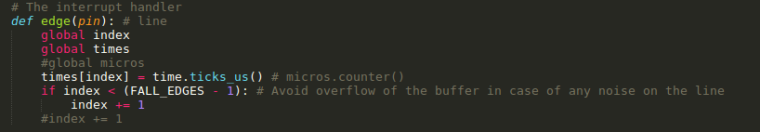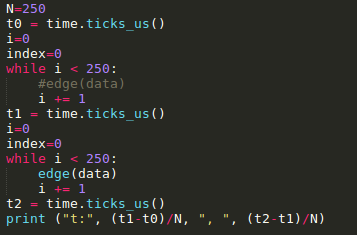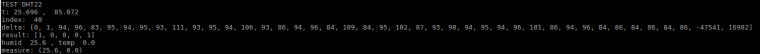# Time & Interrupts

• Dear Developers,

i am currently trying to develop a driver for the DHT11/DHT22 by using
interrupt and callback handlers.
The sensor sends its data by toggling the data pin from high to low.
These flanks are already detected by my code, i can count them and all are
detected.

But i need to measure the time difference between the flanks, a 1
has the length of approx. 130 us and a 0 of approx. 78 us.
When executing the code, the recorded time stamps are all above 130 us,
so they are unusable. Is there any possibility to speed things up here?
I assume that the queuing of interrupts produces those long times.

Here is the result of a measurement:

[120.575, 256.6, 401.2, 547.225, 682.625, 829.225, 973.1, 1109.5, 1255.1, 1402.6, 1548.6, 1681.4, 1826.45, 1979.675, 2122.75, 2265.425, 2389.325, 2535.075, 2668.4, 2802.9, 2957.15, 3092.35, 3227.575, 3360.9, 3505.95, 3640.4, 3786.925, 3925.85, 4060.325, 4184.05, 4307.825, 4431.625, 4555.325, 4679.05, 4802.825, 4934.1, 5057.8, 5181.525, 5305.3, 5429.1, 5552.8, 5676.525]

Here is the code of the handler:

``````def handler(self, arg):
# Increase the index if we are still within the bounds of the array
if(self._samplesIndex < self._MAXSAMPLES - 1):
self._samplesIndex = self._samplesIndex + 1
``````

and here the code to register the handler:

``````self._dataPin.callback(Pin.IRQ_FALLING, self._irqHandler.handler)
``````

Any ideas how to improve the situation?

Marc

• @Emmanuel-Goudot Yes, that's the piece of code below, which works for my DHT22 on a Lopy. It is buried far below in a thread, where two people fight to get it running(https://forum.pycom.io/topic/1172/pure-python-library-for-reading-dht-sensor-on-pycom-boards). No clue what their problem is, since I consider it solved, even if not bullet proof.

``````import time
from machine import enable_irq, disable_irq,  Pin

class DTHResult:
'DHT sensor result returned by DHT.read() method'

ERR_NO_ERROR = 0
ERR_MISSING_DATA = 1
ERR_CRC = 2

error_code = ERR_NO_ERROR
temperature = -1
humidity = -1

def __init__(self, error_code, temperature, humidity):
self.error_code = error_code
self.temperature = temperature
self.humidity = humidity

def is_valid(self):
return self.error_code == DTHResult.ERR_NO_ERROR

class DTH:
'DHT sensor (dht11, dht21,dht22) reader class for Pycom'

__pin = Pin('P3', mode=Pin.OPEN_DRAIN)
__dhttype = 0

def __init__(self, pin, sensor=0):
self.__pin = pin
self.__pin(1)
self.__dhttype = sensor

time.sleep(1)

# send initial high
#self.__send_and_sleep(1, 25000)

# pull down to low
#self.__send_and_sleep(0, 40000)

# collect data into an array
data = self.__collect_input()

# parse lengths of all data pull up periods
pull_up_lengths = self.__parse_data_pull_up_lengths(data)
# if bit count mismatch, return error (4 byte data + 1 byte checksum)
print(pull_up_lengths)
if len(pull_up_lengths) != 40:
return DTHResult(DTHResult.ERR_MISSING_DATA, 0, 0)

# calculate bits from lengths of the pull up periods
bits = self.__calculate_bits(pull_up_lengths)

# we have the bits, calculate bytes
the_bytes = self.__bits_to_bytes(bits)
print(the_bytes)
# calculate checksum and check
checksum = self.__calculate_checksum(the_bytes)
if the_bytes != checksum:
return DTHResult(DTHResult.ERR_CRC, 0, 0)

# ok, we have valid data, return it
[int_rh, dec_rh, int_t, dec_t, csum] = the_bytes
if self.__dhttype==0:		#dht11
rh = int_rh		#dht11 20% ~ 90%
t = int_t	#dht11 0..50°C
else:			#dht21,dht22
rh = ((int_rh * 256) + dec_rh)/10
t = (((int_t & 0x7F) * 256) + dec_t)/10
if (int_t & 0x80) > 0:
t *= -1
return DTHResult(DTHResult.ERR_NO_ERROR, t, rh)

def __send_and_sleep(self, output, mysleep):
self.__pin(output)
time.sleep_us(mysleep)

def __collect_input(self):
# collect the data while unchanged found
unchanged_count = 0
# this is used to determine where is the end of the data
max_unchanged_count = 100
last = -1
data = []
m = *500	   # needs long sample size to grab all the bits from the DHT
self.__pin(0)
time.sleep_us(20000)
self.__pin(1)
irqf = disable_irq()
for i in range(len(m)):
m[i] = self.__pin()      ## sample input and store value
enable_irq(irqf)
for i in range(len(m)):
current = m[i]
data.append(current)
if last != current:
unchanged_count = 0
last = current
else:
unchanged_count += 1
if unchanged_count > max_unchanged_count:
break
#print(data)
return data

def __parse_data_pull_up_lengths(self, data):
STATE_INIT_PULL_DOWN = 1
STATE_INIT_PULL_UP = 2
STATE_DATA_FIRST_PULL_DOWN = 3
STATE_DATA_PULL_UP = 4
STATE_DATA_PULL_DOWN = 5

state = STATE_INIT_PULL_UP

lengths = [] # will contain the lengths of data pull up periods
current_length = 0 # will contain the length of the previous period

for i in range(len(data)):

current = data[i]
current_length += 1

if state == STATE_INIT_PULL_DOWN:
if current == 0:
# ok, we got the initial pull down
state = STATE_INIT_PULL_UP
continue
else:
continue
if state == STATE_INIT_PULL_UP:
if current == 1:
# ok, we got the initial pull up
state = STATE_DATA_FIRST_PULL_DOWN
continue
else:
continue
if state == STATE_DATA_FIRST_PULL_DOWN:
if current == 0:
# we have the initial pull down, the next will be the data pull up
state = STATE_DATA_PULL_UP
continue
else:
continue
if state == STATE_DATA_PULL_UP:
if current == 1:
# data pulled up, the length of this pull up will determine whether it is 0 or 1
current_length = 0
state = STATE_DATA_PULL_DOWN
continue
else:
continue
if state == STATE_DATA_PULL_DOWN:
if current == 0:
# pulled down, we store the length of the previous pull up period
lengths.append(current_length)
state = STATE_DATA_PULL_UP
continue
else:
continue

return lengths

def __calculate_bits(self, pull_up_lengths):
# find shortest and longest period
shortest_pull_up = 1000
longest_pull_up = 0

for i in range(0, len(pull_up_lengths)):
length = pull_up_lengths[i]
if length < shortest_pull_up:
shortest_pull_up = length
if length > longest_pull_up:
longest_pull_up = length

# use the halfway to determine whether the period it is long or short
halfway = shortest_pull_up + (longest_pull_up - shortest_pull_up) / 2
bits = []

for i in range(0, len(pull_up_lengths)):
bit = False
if pull_up_lengths[i] > halfway:
bit = True
bits.append(bit)

return bits

def __bits_to_bytes(self, bits):
the_bytes = []
byte = 0

for i in range(0, len(bits)):
byte = byte << 1
if (bits[i]):
byte = byte | 1
else:
byte = byte | 0
if ((i + 1) % 8 == 0):
the_bytes.append(byte)
byte = 0
#print(the_bytes)
return the_bytes

def __calculate_checksum(self, the_bytes):
return the_bytes + the_bytes + the_bytes + the_bytes & 255

``````

• Hello @robert-hh ,
I guess you have succeeded working with DHT11/DHT22 ?
Could you share python library (that work) ?
Thanks a lot,
Emmanuel.

• @MaSePreetz There was a discussion about pin interrupt latency here: https://forum.pycom.io/topic/936/pin-interrupt-latency
Unless newer version of the firmware improve things drastically, there is no chance to get data from the DHT11/DHT22 with an interrupt using driver. The only Python method that works for me is a busy polling method.

• Hello @livius ,
with such interrupt handler:tested withI get such results:empty loop ~25µs, loop with hadler: ~85µs (handler alone: 65µs ?)
deltas are differences of time.ticks_us() and minimum value I get is ~84µs

Would it be possible to add value of timer inside the object passed to interrupt handler, or as second parameter ???

BR,
Emmanuel.

• Hi Marc,

I've also tried to measure a DHT22's pulse length using an interrupt callbacks. Even with the smallest (and hopefully fastest) interrupt routine I got the same results as you did; I never measured a pulse length below 100 us.

For what I did and the results see here: https://github.com/erikdelange/WiPy-2.0-DHT22

In the end the only thing which worked for me was to use polling, and even then I had to temporarily disable interrupts to get a usable result.

Regards
Erik

• @MaSePreetz

When executing the code, the recorded time stamps are all above 130 us,

You register for IRQ_FALLING, then how you detect how long is 0 and how long is 1?

For IRQ_FALLING you get info that it is changing from 1 to 0. and e.g. it stay in 0 for 50us and it raising to 1 and stay for 100us and then back you get IRQ_FALLING
you count this as 150us but do not know how long is 0 and how long is 1.

EDIT
Ah i forgot that DHT send low 50us and variable length of high like 28 for 0 and 70 for 1
then your concept is good - you should got 78us for 0 or 120us for 1

But for dht better is to Pull pin
and i do not know how long your operation in `handler` take time?